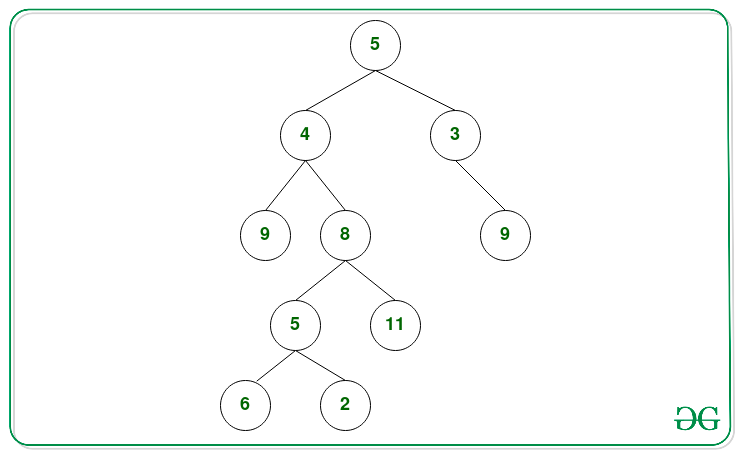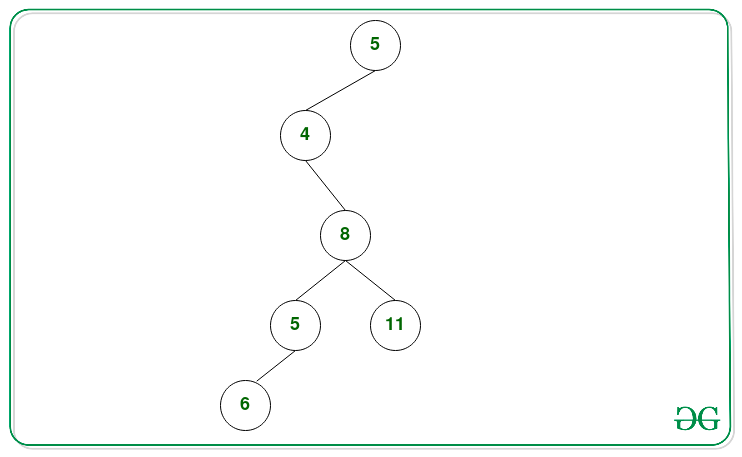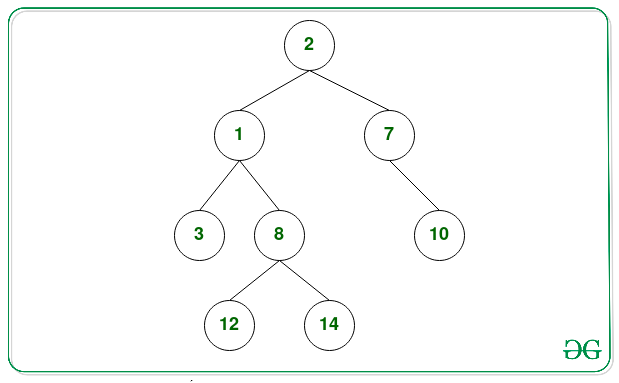# Remove nodes from Binary Tree such that sum of all remaining root-to-leaf paths is atleast K

• Difficulty Level : Medium
• Last Updated : 21 Jun, 2021

Given a Binary Tree and an integer K, the task is to delete nodes from the given Tree such that the sum of all nodes of all remaining root to leaf paths is at least K.

Examples:

Input: K = 27Output: 5 4 8 5 6 11
Explanation:
Below are the paths whose sum is less than 27:

• 5 -> 3 -> 9: Path Sum = 5 + 3 + 9 = 17.
• 5 -> 4 -> 9: Path Sum = 5 + 4 + 9 = 18.
• 5 -> 4 -> 8 -> 5 -> 2: Path Sum = 5 + 4 + 8 + 5 + 2 = 24.

Below is the tree after deleting the required nodes that such that sum of all paths is at least 27:Input: K = 10Output: 2 1 8 12 14 7 10

Approach: The idea is to use recursion and perform the Postorder Traversal and delete those nodes whose addition to the path sum is less than K. Below are the steps:

• Perform the Post Order Traversal on the given Tree and during this traversal pass the sum of all nodes from the root node to each node.
• During traversal, if we reach the leaf node then check if the sum of all nodes till that node is less than K?. If found to be true, remove that node by returning the NULL node from that node.
• Repeat the above step for every leaf node encounters in the update tree.
• After the above steps, print the Preorder Traversal of the modified Tree.

Below is the implementation of the above approach:

## C++

 `// C++ program for the above approach` `#include ` `using` `namespace` `std;`   `// Tree Node Class` `struct` `Node ` `{` `    ``int` `data;` `    ``Node *left;` `    ``Node *right;`   `    ``Node(``int` `x)` `    ``{` `        ``data = x;` `        ``left = right = NULL;` `    ``}` `};`   `// Utility function that deletes nodes` `// from the Tree such that every root` `// to leaf path sum is at least K` `Node *removePathLessThanK(Node *node, ``int` `K,` `                          ``int` `sum)` `{` `    `  `    ``// Base Case` `    ``if` `(node == NULL) ` `    ``{` `        ``return` `NULL;` `    ``}`   `    ``// Recurse to the left` `    ``if` `(node->left != NULL)` `    ``{` `        ``node->left = removePathLessThanK(` `                     ``node->left, K,` `                     ``sum + node->left->data);` `    ``}`   `    ``// Recurse to the right` `    ``if` `(node->right != NULL)` `    ``{` `        ``node->right = removePathLessThanK(` `                      ``node->right, K,` `                      ``sum + node->right->data);` `    ``}`   `    ``// Check path sum at leaf node` `    ``// is lesser than K, return NULL` `    ``if` `(node->left == NULL && ` `        ``node->right == NULL && sum < K)` `    ``{` `        ``node = NULL;` `        ``return` `node;` `    ``}`   `    ``// Otherwise return the` `    ``// current node as it is` `    ``return` `node;` `}`   `// Function to print the preorder` `// traversal of the Tree` `void` `viewTree(Node *node)` `{` `    `  `    ``// If node is not NULL` `    ``if` `(node != NULL) ` `    ``{` `        `  `        ``// Print the node` `        ``cout << node->data << ``" "``;`   `        ``// Left and Right Traversal` `        ``viewTree(node->left);` `        ``viewTree(node->right);` `    ``}` `}`   `// Function that deletes the nodes` `// from the Tree such that every root` `// to leaf path sum is at least K` `void` `removePathLessThanKUtil(Node *node, ``int` `K,` `                             ``int` `sum)` `{` `    `  `    ``// Function Call to delete Nodes` `    ``Node *result = removePathLessThanK(node, K, sum);` `    `  `    ``// Preorder Traversal of the` `    ``// modified Tree` `    ``viewTree(result);` `}`   `// Driver Code` `int` `main()` `{` `    `  `    ``// Given sum K` `    ``int` `K = 27;`   `    ``// Given Binary Tree` `    ``Node *root = NULL;` `    ``root = ``new` `Node(5);` `    ``root->right = ``new` `Node(3);` `    ``root->left = ``new` `Node(4);` `    ``root->left->left = ``new` `Node(9);` `    ``root->right->right = ``new` `Node(9);` `    ``root->left->right = ``new` `Node(8);` `    ``root->left->right->right = ``new` `Node(11);` `    ``root->left->right->left = ``new` `Node(5);` `    ``root->left->right->left->left = ``new` `Node(6);` `    ``root->left->right->left->right = ``new` `Node(2);` `    ``root->right->right->right = ``new` `Node(4);`   `    ``// Function Call` `    ``removePathLessThanKUtil(root, K, root->data);` `}`   `// This code is contributed by mohit kumar 29`

## Java

 `// Java program for the above approach`   `import` `java.io.*;` `import` `java.util.*;`   `// Tree Node Class` `class` `Node {` `    ``int` `data;` `    ``Node left;` `    ``Node right;` `}`   `class` `path {`   `    ``// Function to insert node in the` `    ``// given Binary Tree` `    ``public` `Node insert(``int` `val)` `    ``{` `        ``Node n = ``new` `Node();` `        ``n.data = val;` `        ``n.left = ``null``;` `        ``n.right = ``null``;` `        ``return` `n;` `    ``}`   `    ``// Utility function that deletes nodes` `    ``// from the Tree such that every root` `    ``// to leaf path sum is at least K` `    ``public` `Node removePathLessThanK(` `        ``Node node, ``int` `K, ``int` `sum)` `    ``{` `        ``// Base Case` `        ``if` `(node == ``null``) {` `            ``return` `null``;` `        ``}`   `        ``// Recurse to the left` `        ``if` `(node.left != ``null``) {` `            ``node.left` `                ``= removePathLessThanK(` `                    ``node.left, K,` `                    ``sum + node.left.data);` `        ``}`   `        ``// Recurse to the right` `        ``if` `(node.right != ``null``) {` `            ``node.right` `                ``= removePathLessThanK(` `                    ``node.right, K,` `                    ``sum + node.right.data);` `        ``}`   `        ``// Check path sum at leaf node` `        ``// is lesser than K, return NULL` `        ``if` `(node.left == ``null` `            ``&& node.right == ``null` `            ``&& sum < K) {` `            ``node = ``null``;` `            ``return` `node;` `        ``}`   `        ``// Otherwise return the` `        ``// current node as it is` `        ``return` `node;` `    ``}`   `    ``// Function to print the preorder` `    ``// traversal of the Tree` `    ``public` `void` `viewTree(Node node)` `    ``{` `        ``// If node is not NULL` `        ``if` `(node != ``null``) {`   `            ``// Print the node` `            ``System.out.print(node.data + ``" "``);`   `            ``// Left and Right Traversal` `            ``viewTree(node.left);` `            ``viewTree(node.right);` `        ``}` `    ``}`   `    ``// Function that deletes the nodes` `    ``// from the Tree such that every root` `    ``// to leaf path sum is at least K` `    ``public` `void` `removePathLessThanKUtil(` `        ``Node node, ``int` `K, ``int` `sum)` `    ``{` `        ``// Function Call to delete Nodes` `        ``Node result = removePathLessThanK(` `            ``node, K, sum);`   `        ``// Preorder Traversal of the` `        ``// modified Tree` `        ``viewTree(result);` `    ``}` `}`   `// Driver Code` `class` `GFG {`   `    ``// Driver Code` `    ``public` `static` `void` `main(String[] args)` `    ``{` `        ``// Given sum K` `        ``int` `K = ``27``;`   `        ``// Object of class path` `        ``path b = ``new` `path();`   `        ``// Given Binary Tree` `        ``Node root = ``null``;` `        ``root = b.insert(``5``);` `        ``root.right = b.insert(``3``);` `        ``root.left = b.insert(``4``);` `        ``root.left.left = b.insert(``9``);` `        ``root.right.right = b.insert(``9``);` `        ``root.left.right = b.insert(``8``);` `        ``root.left.right.right = b.insert(``11``);` `        ``root.left.right.left = b.insert(``5``);` `        ``root.left.right.left.left = b.insert(``6``);` `        ``root.left.right.left.right = b.insert(``2``);` `        ``root.right.right.right = b.insert(``4``);`   `        ``// Function Call` `        ``b.removePathLessThanKUtil(` `            ``root, K, root.data);` `    ``}` `}`

## Python3

 `# Python3 program for the above approach`   `# Tree Node Class` `class` `newNode:` `    `  `    ``def` `__init__(``self``, x):` `        `  `        ``self``.data ``=` `x` `        ``self``.left ``=` `None` `        ``self``.right ``=` `None`   `# Utility function that deletes nodes` `# from the Tree such that every root` `# to leaf path sum is at least K` `def` `removePathLessThanK(node, K, ``sum``):` `    `  `    ``# Base Case` `    ``if` `(node ``=``=` `None``):` `        ``return` `None`   `    ``# Recurse to the left` `    ``if` `(node.left !``=` `None``):` `        ``node.left ``=` `removePathLessThanK(` `                    ``node.left, K, ` `                    ``sum` `+` `node.left.data)`   `    ``# Recurse to the right` `    ``if` `(node.right !``=` `None``):` `        ``node.right ``=` `removePathLessThanK(` `                     ``node.right, K,` `                     ``sum` `+` `node.right.data)`   `    ``# Check path sum at leaf node` `    ``# is lesser than K, return None` `    ``if` `(node.left ``=``=` `None` `and` `       ``node.right ``=``=` `None` `and` `sum` `< K):` `        ``node ``=` `None` `        ``return` `node`   `    ``# Otherwise return the` `    ``# current node as it is` `    ``return` `node`   `# Function to print the preorder` `# traversal of the Tree` `def` `viewTree(node):` `    `  `    ``# If node is not None` `    ``if` `(node !``=` `None``):` `        `  `        ``# Print the node` `        ``print``(node.data, end ``=` `" "``)`   `        ``# Left and Right Traversal` `        ``viewTree(node.left)` `        ``viewTree(node.right)`   `# Function that deletes the nodes` `# from the Tree such that every root` `# to leaf path sum is at least K` `def` `removePathLessThanKUtil(node, K, ``sum``):` `    `  `    ``# Function Call to delete Nodes` `    ``result ``=` `removePathLessThanK(node, K, ``sum``)` `    `  `    ``# Preorder Traversal of the` `    ``# modified Tree` `    ``viewTree(result)`   `# Driver Code` `if` `__name__ ``=``=` `'__main__'``:` `    `  `    ``# Given sum K` `    ``K ``=` `27`   `    ``# Given Binary Tree` `    ``root ``=` `None` `    ``root ``=` `newNode(``5``)` `    ``root.right ``=` `newNode(``3``)` `    ``root.left ``=` `newNode(``4``)` `    ``root.left.left ``=` `newNode(``9``)` `    ``root.right.right ``=` `newNode(``9``)` `    ``root.left.right ``=` `newNode(``8``)` `    ``root.left.right.right ``=` `newNode(``11``)` `    ``root.left.right.left ``=` `newNode(``5``)` `    ``root.left.right.left.left ``=` `newNode(``6``)` `    ``root.left.right.left.right ``=` `newNode(``2``)` `    ``root.right.right.right ``=` `newNode(``4``)`   `    ``# Function Call` `    ``removePathLessThanKUtil(root, K, root.data)`   `# This code is contributed by SURENDRA_GANGWAR`

## C#

 `// C# program for the above approach` `using` `System;`   `// Tree Node Class` `public` `class` `Node ` `{` `    ``public` `int` `data;` `    ``public` `Node left;` `    ``public` `Node right;` `}`   `class` `path{`   `// Function to insert node in the` `// given Binary Tree` `public` `Node insert(``int` `val)` `{` `    ``Node n = ``new` `Node();` `    ``n.data = val;` `    ``n.left = ``null``;` `    ``n.right = ``null``;` `    ``return` `n;` `}`   `// Utility function that deletes nodes` `// from the Tree such that every root` `// to leaf path sum is at least K` `public` `Node removePathLessThanK(Node node, ` `                                ``int` `K, ``int` `sum)` `{` `    `  `    ``// Base Case` `    ``if` `(node == ``null``)` `    ``{` `        ``return` `null``;` `    ``}`   `    ``// Recurse to the left` `    ``if` `(node.left != ``null``)` `    ``{` `        ``node.left = removePathLessThanK(` `                    ``node.left, K,` `                    ``sum + node.left.data);` `    ``}`   `    ``// Recurse to the right` `    ``if` `(node.right != ``null``)` `    ``{` `        ``node.right = removePathLessThanK(` `                     ``node.right, K,` `                     ``sum + node.right.data);` `    ``}`   `    ``// Check path sum at leaf node` `    ``// is lesser than K, return NULL` `    ``if` `(node.left == ``null` `&& ` `       ``node.right == ``null` `&& sum < K)` `    ``{` `        ``node = ``null``;` `        ``return` `node;` `    ``}`   `    ``// Otherwise return the` `    ``// current node as it is` `    ``return` `node;` `}`   `// Function to print the preorder` `// traversal of the Tree` `public` `void` `viewTree(Node node)` `{` `    `  `    ``// If node is not NULL` `    ``if` `(node != ``null``)` `    ``{` `        `  `        ``// Print the node` `        ``Console.Write(node.data + ``" "``);`   `        ``// Left and Right Traversal` `        ``viewTree(node.left);` `        ``viewTree(node.right);` `    ``}` `}`   `// Function that deletes the nodes` `// from the Tree such that every root` `// to leaf path sum is at least K` `public` `void` `removePathLessThanKUtil(Node node, ` `                                    ``int` `K, ``int` `sum)` `{` `    `  `    ``// Function Call to delete Nodes` `    ``Node result = removePathLessThanK(` `        ``node, K, sum);`   `    ``// Preorder Traversal of the` `    ``// modified Tree` `    ``viewTree(result);` `}` `}`   `// Driver Code` `class` `GFG{`   `// Driver Code` `public` `static` `void` `Main(String[] args)` `{` `    `  `    ``// Given sum K` `    ``int` `K = 27;`   `    ``// Object of class path` `    ``path b = ``new` `path();`   `    ``// Given Binary Tree` `    ``Node root = ``null``;` `    ``root = b.insert(5);` `    ``root.right = b.insert(3);` `    ``root.left = b.insert(4);` `    ``root.left.left = b.insert(9);` `    ``root.right.right = b.insert(9);` `    ``root.left.right = b.insert(8);` `    ``root.left.right.right = b.insert(11);` `    ``root.left.right.left = b.insert(5);` `    ``root.left.right.left.left = b.insert(6);` `    ``root.left.right.left.right = b.insert(2);` `    ``root.right.right.right = b.insert(4);`   `    ``// Function Call` `    ``b.removePathLessThanKUtil(` `        ``root, K, root.data);` `}` `}`   `// This code is contributed by Princi Singh`

## Javascript

 ``

Output:

`5 4 8 5 6 11`

Time Complexity: O(N), where N is the number of nodes in the given Tree.
Auxiliary Space: O(N)

My Personal Notes arrow_drop_up
Recommended Articles
Page :